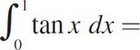# AP Calculus AB Practice Test 8

### Test Information10 questions20 minutes

Calculator Disallowed

1. If f(x) = sin2x, Find f′″(x).

2. Find the slope of the normal line to y = x + cos xy at (0, 1).

3.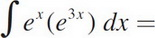4.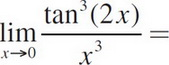5. A solid is generated when the region in the first quadrant bounded by the graph of y = 1 + sin2x, the line x =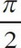, the x-axis, and the y-axis is revolved about the x-axis. Its volume is found by evaluating which of the following integrals?

6. If y =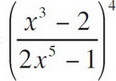, find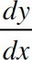at x = 1.

7.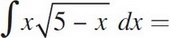8. If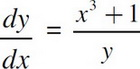= and y = 2 when x =1, then, when x = 2, y =

9. The graph of y = 5x4 - x5 has an inflection point (or points) at

10.